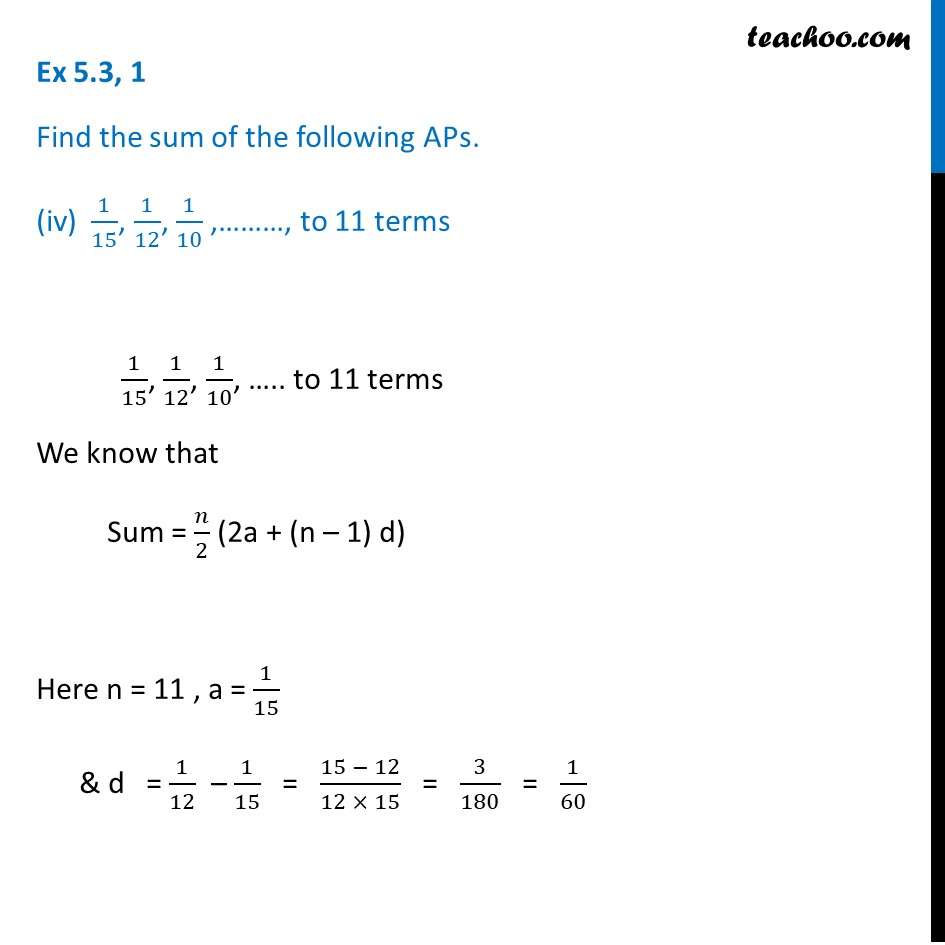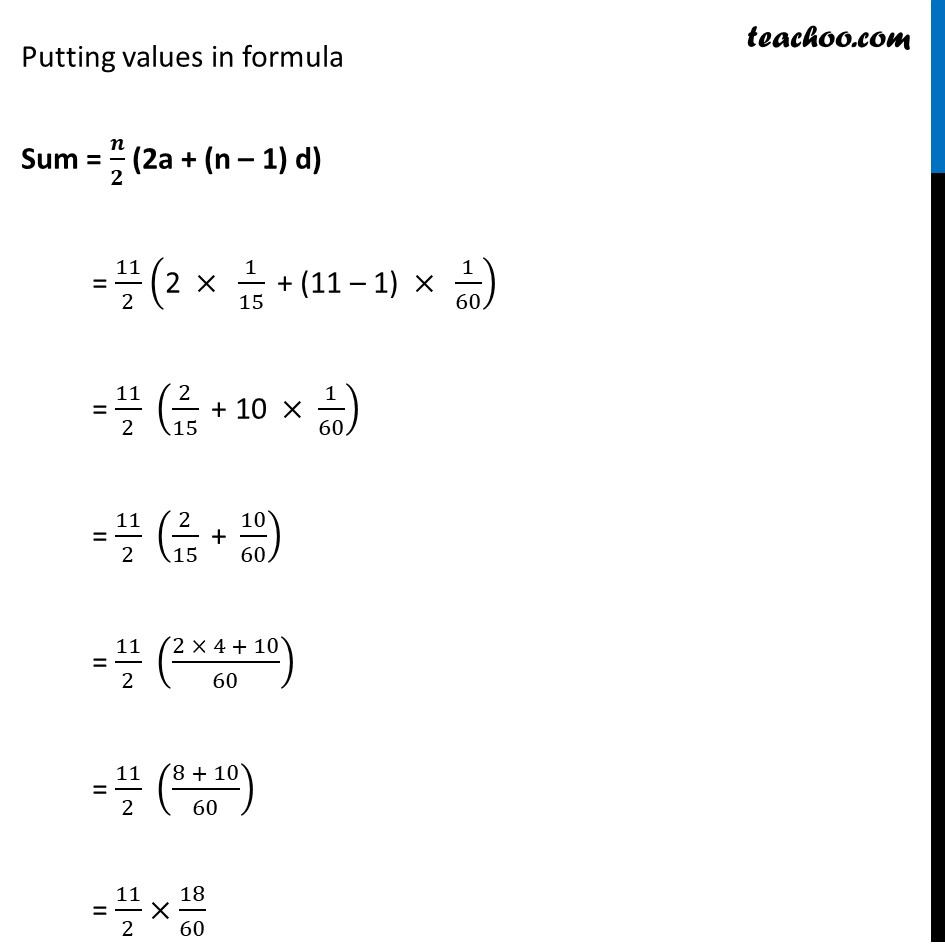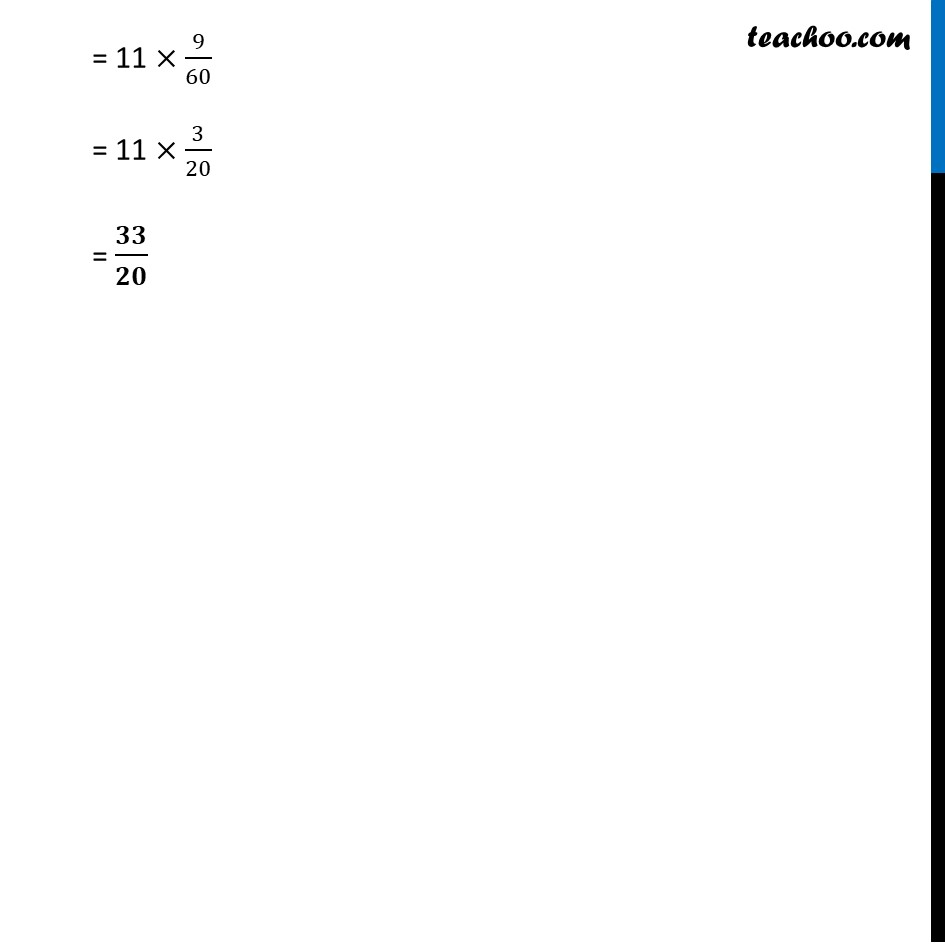Ex 5.3

Chapter 5 Class 10 Arithmetic Progressions (Term 2)
Serial order wise### Transcript

Ex 5.3, 1 Find the sum of the following APs. (iv) 1/15, 1/12, 1/10 ,………, to 11 terms 1/15, 1/12, 1/10, ….. to 11 terms We know that Sum = 𝑛/2 (2a + (n – 1) d) Here n = 11 , a = 1/15 & d = 1/12 – 1/15 = (15 − 12)/(12 × 15) = 3/180 = 1/60 Putting values in formula Sum = 𝒏/𝟐 (2a + (n – 1) d) = 11/2 ("2 " ×" " 1/15 " + (11 – 1) " ×" " 1/60) = 11/2 (2/15 " + 10 " × 1/60) = 11/2 (2/15 " + " 10/60) = 11/2 ((2 × 4 + 10)/60) = 11/2 ((8 + 10)/60) = 11/2 × 18/60 = 11 × 9/60 = 11 × 3/20 = 𝟑𝟑/𝟐𝟎